# Finance Formulae

A compiled listing of the most common and useful fiance formulas.
Subject: Finance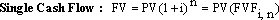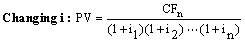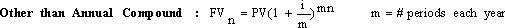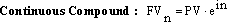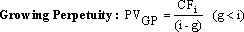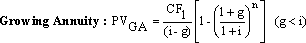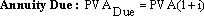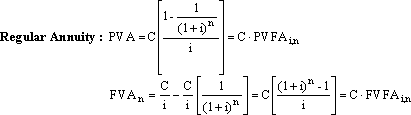NPV = PVinflows – PVoutflows
IRR = i that makes NPV = 0
UAS – annuity that = PV of irregular cash flow pattern

Ri= realized asset = expected return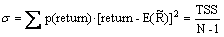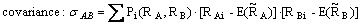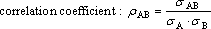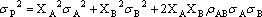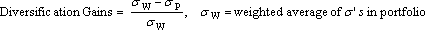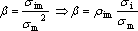ß=1 ⇒ average risk
ß<1 ⇒ below average risk

ß>1 ⇒ above average risk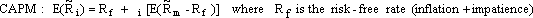CAPM relates a security’s relevant risk (ß) to expected return.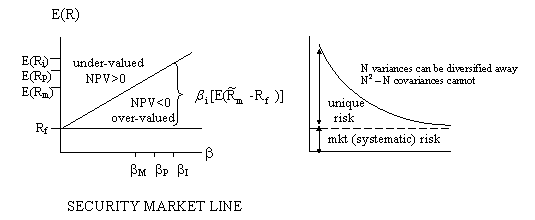CFAT (indirect) = market value of asset sold – tax liability = MV – (MV – BV)(𝜏)
Increase in working capital, ΔWC = CA – CL
ΔCFAT = (ΔS – ΔC – ΔD)(1 – 𝜏) + ΔD {S = sales, C = costs, D = depreciation}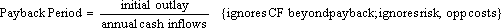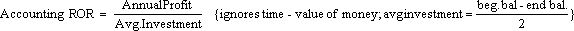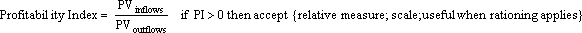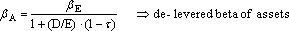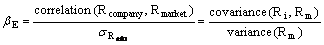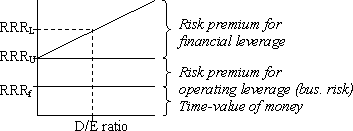NPV (loan) = Amount borrowed – PV (all after-tax payments)

APV (Adjusted PV) = All equity value – PV(FC) + NPV(loan)

1. Calculate NPV for all-equity financing
2. Adjust NPV for flotation costs
3. Adjust NPV for tax shield + subsidization

WACC (weighted average cost of capital)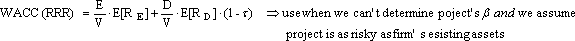{D + E = V}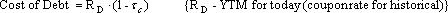Pure Play Method

2. to proposed project.

3. Determine equity beta (ße) for pre-play firm’s stock.
4. Calculate pure-play assets ßA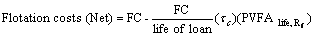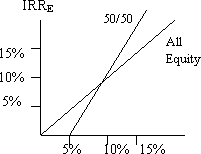Loan \$ = Gross Proceeds (1 – FC%)

When to Lease?
If there is a tax differential or if the lease lowers transaction costs and/or reduces uncertainty

Lessee (user): 0 = Io – Max[1-𝜏cu] × PVFA[T,rD(1-𝜏cu)] – D × 𝜏cu × PVFA[T,rD(1-𝜏cu)] Lessor (owner): 0 = -Io – Min[1-𝜏co] × PVFA[T,rD(1-𝜏co)] + D × 𝜏co × PVFA[T,rD(1-𝜏co)]

RRRD = Rf + ßD[Rm – Rf] RRRA = Rf + ßA[Rm – Rf] ROE = ROA + D/E[ROA – Rf] ROA = RRREu = Rf + ßA[Rm – Rf] – expected return on an un-levered firm’s assets
RRREl = RRREu + D/E [RRREu – RRRD] ROA = [E/(D+E)](ROE)  + [D/(D+E)](Rf)
ßA = [D/(D+E)]( bD)  + [E/(D+E)]( ßE)
ßE = ßA + D/E(ßA – ßD)
E(RE) = Rf + ßE  [E(Rm) – Rf] – M&M CAPM (Equity)

Payout Ratio = % Earnings paid as dividends
Retention Ratio = 1 – Payout Ratio
g = (Retention Ratio)(ROE)
ROE = NI/Earnings = Earnings/Book Equity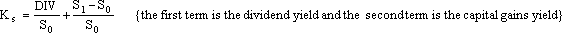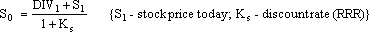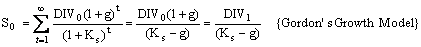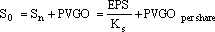PVGO = PV[PV(cash inflows at time t) – PV(cash outflows at time t)] – present value growth option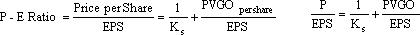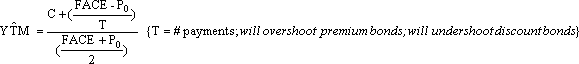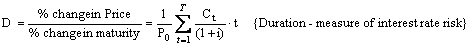Valuefirm = Cost of Assets + PV(OCF)
E = VF – D {D = amount borrowed/rD}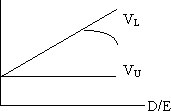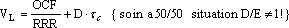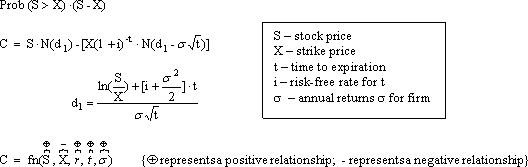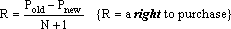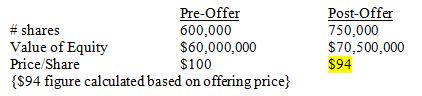1 share offered for \$70 + 4 rights {150,000 new shares offered ⇒ \$10,500,000 additional equity}
⇒ 4 rights = \$94 – \$70 = \$24
⇒ 1 right = \$6

M&M (Modigliani and Miller)
Proposition 1: method of structuring D/E has no effect on firm value
Proposition 2: cost of equity capital increases as amount of debt increases

Assumes:

1. No taxes
2. No transaction costs
3. No changes in investment policy